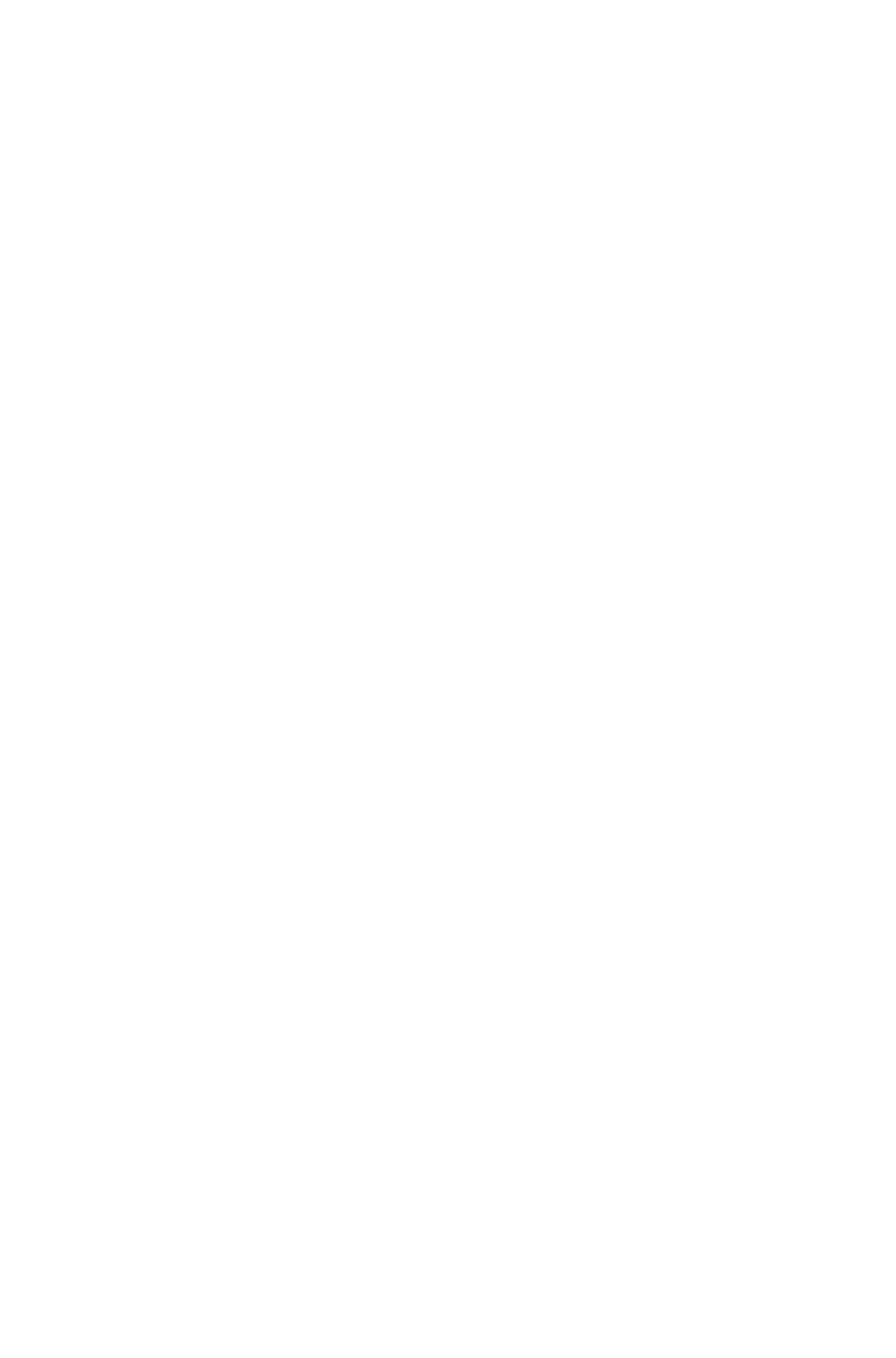# 3.2.3 Total Internal Reflection

### How does Total Internal Reflection Occur?

• When light is moving from a denser medium towards a less dense one, most of the light is refracted, but a small amount of it can be internally reflected
• However, if the angle of the light is great enough then instead of being refracted, ALL of the light is reflected
• This is called Total Internal reflection and happens when the angle of the incident ray is greater than the critical angle for that material
• Two conditions are necessary for total internal reflection to occur:
• The light must be going from a more dense medium towards a less dense one
• The angle of incidence must be greater than the critical angle

#### Exam Tip

If asked to name the phenomena make sure you give the whole name – Total Internal Reflection.

Remember: Total Internal Reflection occurs when going from more dense to less dense and ALL of the light is reflected.

If asked to explain what is meant by the critical angle, you can draw the diagram above (showing the three semi-circular blocks).

Extended Only

### Refractive Index & Critical Angle Equation

• The critical angle, c, of a material is related to its refractive index, n
• The relationship between the two quantities is given by the equation:

#### Exam Tip

When calculating the value of the critical angle using the above equation:

• First use the refractive index, n, to find sin c
• Then use the inverse sin function (sin-1) to find the value of i
Extended Only

### Optical Fibres

• Total internal reflection is also used to reflect light along optical fibres, allowing the high-speed transmission of data on the internet
• Additionally, optical fibres can be used in medicine in order to see within the human bodyDiagram showing the application of total internal reflection in medicine (using an endoscope)

#### Exam Tip

When drawing light reflecting down an optical fibre, make sure that each time it reflects the angle of reflection is equal to the angle of incidence.### Author: Jenna

Jenna studied at Cardiff University before training to become a science teacher at the University of Bath specialising in Biology (although she loves teaching all three sciences at GCSE level!). Teaching is her passion, and with 10 years experience teaching across a wide range of specifications – from GCSE and A Level Biology in the UK to IGCSE and IB Biology internationally – she knows what is required to pass those Biology exams.
CloseClose